# Resultant

(diff) ← Older revision | Latest revision (diff) | Newer revision → (diff)

of two polynomialsandThe element of the fielddefined by the formula: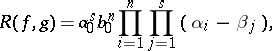(1)

whereis the splitting field of the polynomial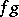(cf. Splitting field of a polynomial), and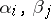are the roots (cf. Root) of the polynomials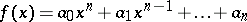and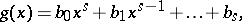respectively. If, then the polynomials have a common root if and only if the resultant equals zero. The following equality holds: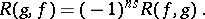The resultant can be written in either of the following ways:(2)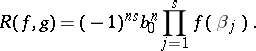(3)

The expressions (1)–(3) are inconvenient for computing the resultant, since they contain the roots of the polynomials. Using the coefficients of the polynomials, the resultant can be expressed in the form of the following determinant of order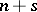: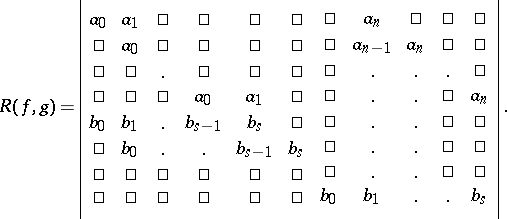(4)

This determinant contains in the firstrows the coefficients of the polynomial, and in the lastrows the coefficients of the polynomial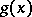, and in the free spaces there are zeros.

The resultant of two polynomialsandwith numerical coefficients can be represented in the form of a determinant of order(or). For this one has to find the remainders from the division ofby,. Let these be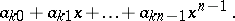ThenThe discriminant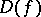of the polynomial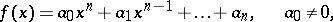can be expressed by the resultant of the polynomialand its derivative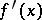in the following way:## Application to solving a system of equations.

Let there be given a system of two algebraic equations with coefficients from a field:(5)

The polynomialsandare written as polynomials in: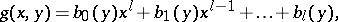and according to formula (4) the resultant of these polynomials (as polynomials in) is calculated. This yields a polynomial that depends only on: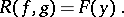One says that the polynomialis obtained by eliminatingfrom the polynomialsand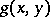. If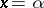andis a solution of the system (5), then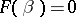, and, conversely, if, then either the polynomials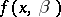or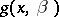have a common root (which must be looked for among the roots of their greatest common divisor), or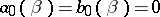. Solving system (5) is thereby reduced to the computation of the roots of the polynomial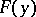and of the common roots of the polynomialsandin one indeterminate.

By analogy, systems of equations with any number of unknowns can be solved; however, this problem leads to extremely cumbersome calculations (see also Elimination theory).

How to Cite This Entry:
Resultant. Encyclopedia of Mathematics. URL: http://encyclopediaofmath.org/index.php?title=Resultant&oldid=15841
This article was adapted from an original article by I.V. Proskuryakov (originator), which appeared in Encyclopedia of Mathematics - ISBN 1402006098. See original article